# How to setup Competitive Programming in Visual Studio Code for C++

GCC compiler installation

We need to install GCC compilers for Windows. Linux has already GCC installed.

Below are the steps for installation:

2. Open Control Panel in your system and then select: System (Control Panel)
3. Click on the Advanced system settings as shown below: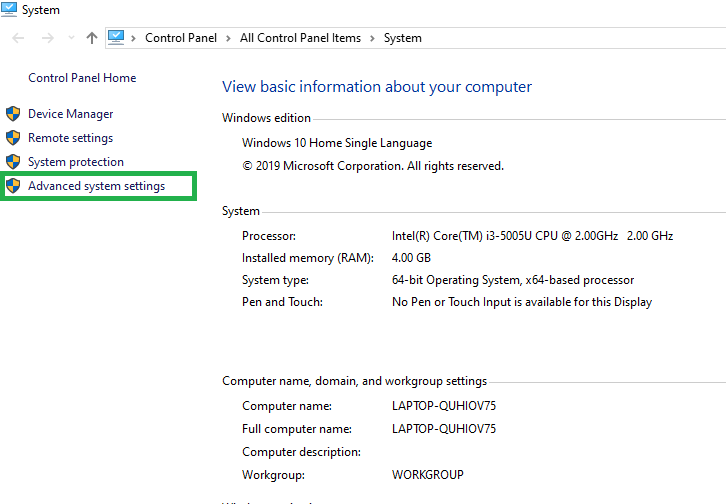4. Click on Environment Variables. In the section System Variables, find the PATH environment variable and select it. Click Edit. If the PATH environment variable does not exist, click New as shown below: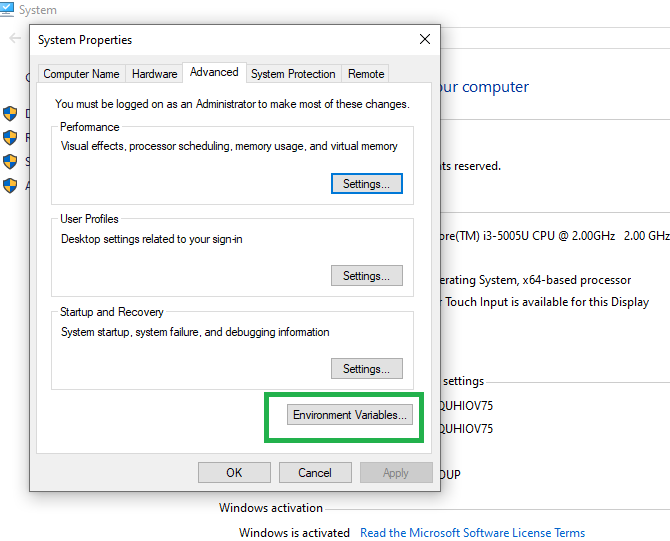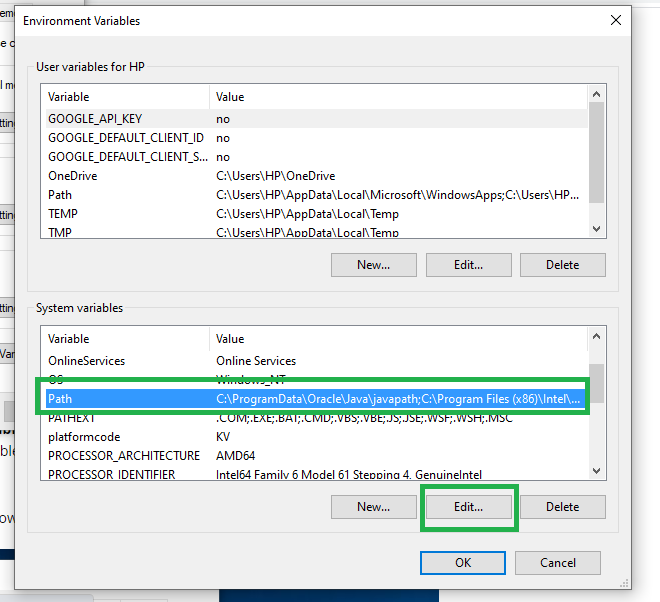5. In the Edit System Variable (or New System Variable) window, specify the value of the PATH environment variable.
6. Copy “C:\MinGW\bin” as shown below: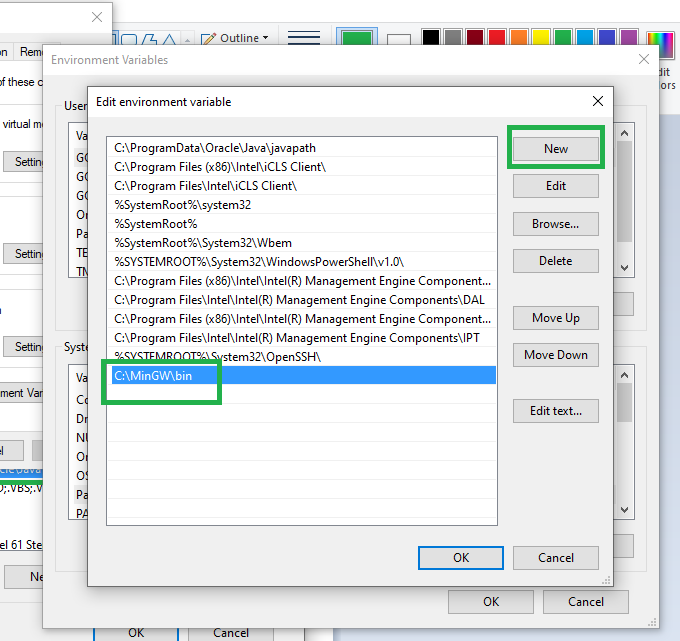7. Click OK.
8. Close all remaining windows by clicking OK.

Setup Template for Competetive Programming

Below is the example for the template for competitive programming which programmers use. You can change this template according to your choice and preference:

## C++

 `// Template for Competitive Programming ` ` `  `#include ` `using` `namespace` `std; ` ` `  `#define fo(i, n) for (i = 0; i < n; i++) ` `#define si(x) scanf("%d", &x) ` `#define sl(x) scanf("%lld", &x) ` `#define ss(s) scanf("%s", s) ` `#define pi(x) printf("%d\n", x) ` `#define pl(x) printf("%lld\n", x) ` `#define ps(s) printf("%s\n", s) ` `#define pb push_back ` `#define mp make_pair ` `#define F first ` `#define S second ` `#define all(x) x.begin(), x.end() ` `#define clr(x) memset(x, 0, sizeof(x)) ` `#define sortall(x) sort(all(x)) ` `typedef` `pair<``int``, ``int``> pii; ` `typedef` `pair pl; ` `typedef` `vector<``int``> vi; ` `typedef` `vector vl; ` `typedef` `vector vpii; ` ` `  `// Drive Code ` `int` `main() ` `{ ` `    ``// Fast Input/Output ` `    ``ios_base::sync_with_stdio(0); ` `    ``cin.tie(0); ` `    ``cout.tie(0); ` ` `  `    ``// Test Cases ` `    ``int` `t = 1; ` `    ``cin >> t; ` `    ``deb(t); ` ` `  `    ``while` `(t--) { ` `    ``} ` ` `  `    ``return` `0; ` `} `

Steps to insert the template code while creating a new .cpp files

• Open VS Code and go to:
`File > Preferences > User Snippets`

and

```Click on New Snippets
```
• Replace the existing code within the braces with the code written below and save it with some name.

• STEP 1: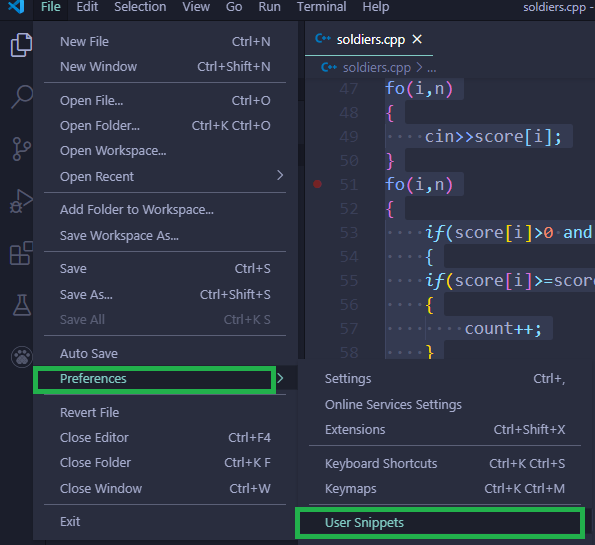• STEP 2: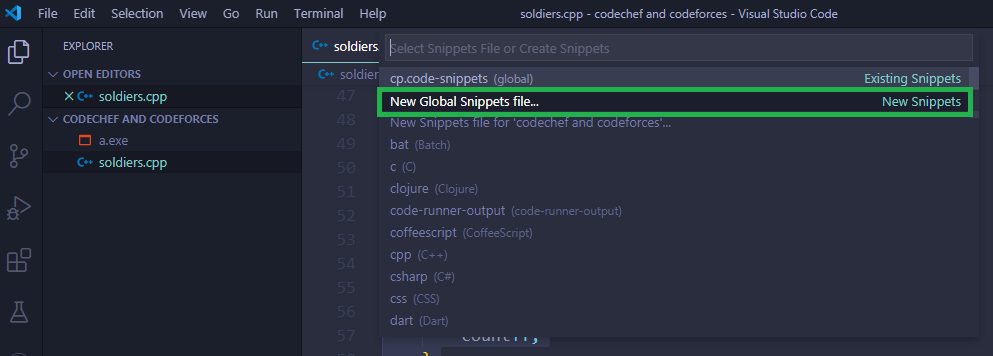• STEP 3: Enter any name as per your choice in the dialouge box.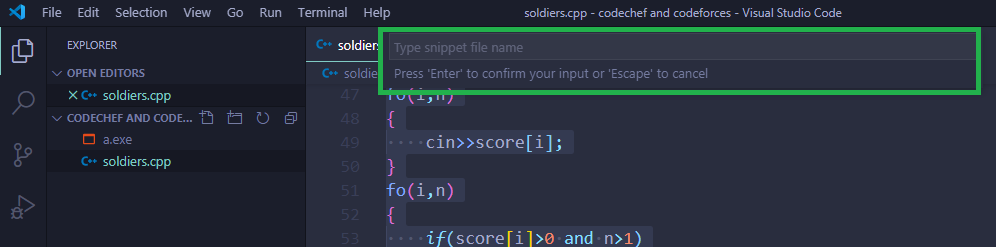• STEP 4: Erase all the commented lines and delete them.Don’t remove the curly braces.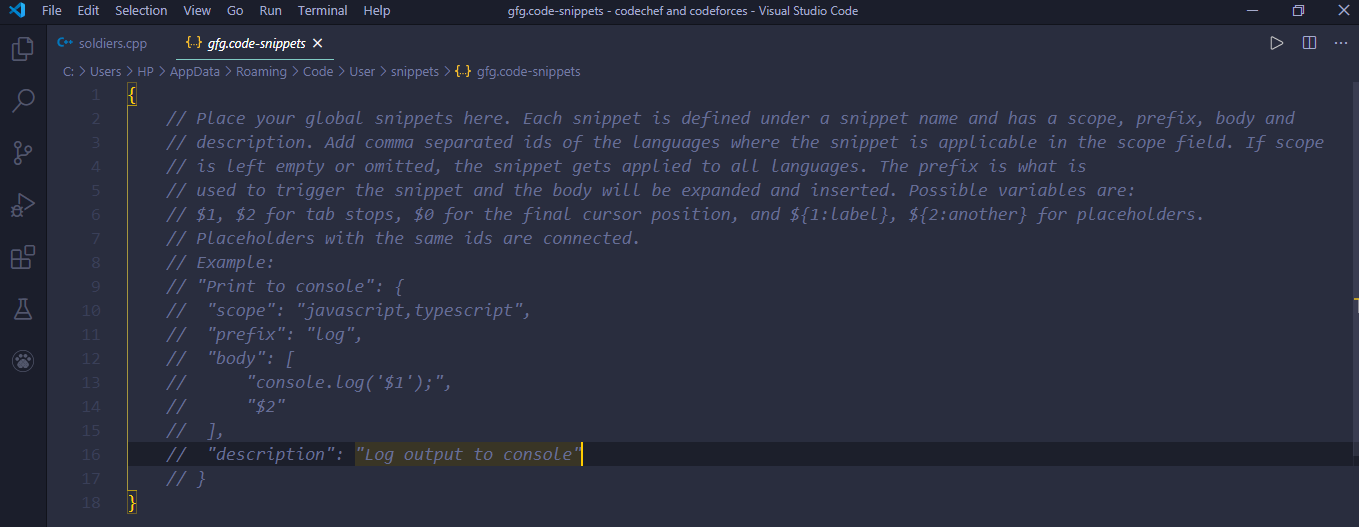• STEP 5: Copy this entire json file from below and paste it in between starting and closing braces.

Note: In Prefix you have to use your personal choice word, here for example i am using gfg. This is very important because it is the key to call the template when you will need this template.

“”: {
“prefix”: “gfg”,
“body”: [
“#include <bits/stdc++.h>”,
“using namespace std;”,
“#define fo(i, n) for (i = 0; i < n; i++)”,
“#define Fo(i, k, n) for (i = k; k < n ? i < n : i > n; k < n ? i += 1 : i -= 1)”,
“#define ll long long”,
“#define si(x) scanf(\”%d\”, &x)”,
“#define sl(x) scanf(\”%lld\”, &x)”,
“#define ss(s) scanf(\”%s\”, s)”,
“#define pi(x) printf(\”%d\\n\”, x)”,
“#define pl(x) printf(\”%lld\\n\”, x)”,
“#define ps(s) printf(\”%s\\n\”, s)”,
“#define deb(x) cout << #x << \”=\” << x << endl”,
“#define deb2(x, y) cout << #x << \”=\” << x << \”, \” << #y << \”=\” << y << endl”,
“#define pb push_back”,
“#define mp make_pair”,
“#define F first”,
“#define S second”,
“#define all(x) x.begin(), x.end()”,
“#define clr(x) memset(x, 0, sizeof(x))”,
“#define sortall(x) sort(all(x))”,
“#define tr(it, a) for (auto it = a.begin(); it != a.end(); it++)”,
“#define PI 3.1415926535897932384626”,
“typedef pair<int, int> pii;”,
“typedef pair<ll, ll> pl;”,
“typedef vector<int> vi;”,
“typedef vector<ll> vl;”,
“typedef vector<pii> vpii;”,
“”,
“”,
“int main()”,
“{“,
” ios_base::sync_with_stdio(0), cin.tie(0), cout.tie(0);”,
” srand(chrono::high_resolution_clock::now().time_since_epoch().count());”,
“”,
” int t = 1;”,
” cin >> t;”,
” deb(t);”,
“”,
” while (t–) {“,
” “,
” }”,
“”,
” return 0;”,
“}”
],
“description”: “”
}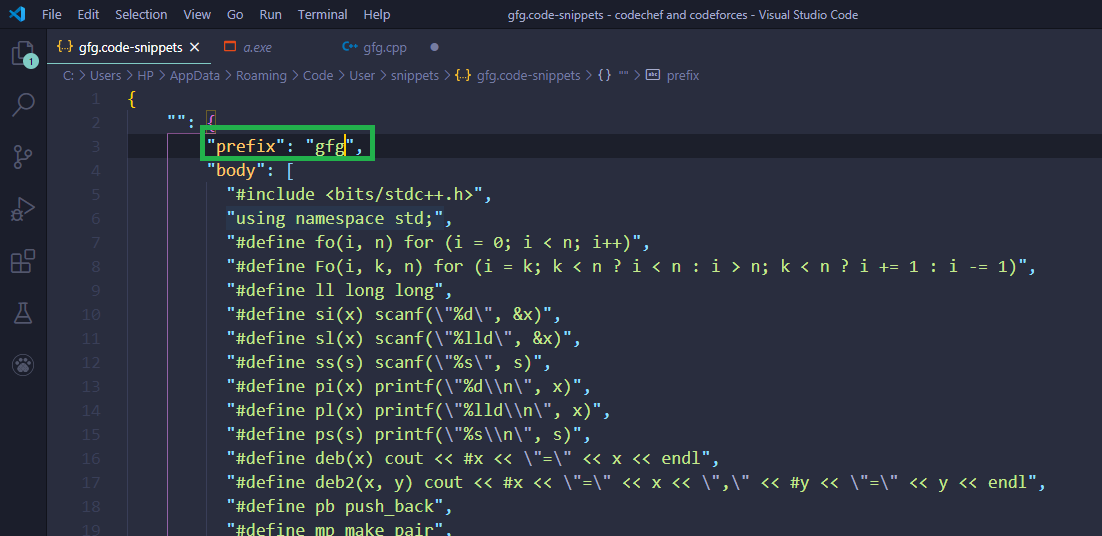• STEP 6: Now Save this by pressing CTRL+S.
• STEP 7: Open a new cpp file and write your prefix or template name that you wrote in the prefix box.(Here i had used gfg).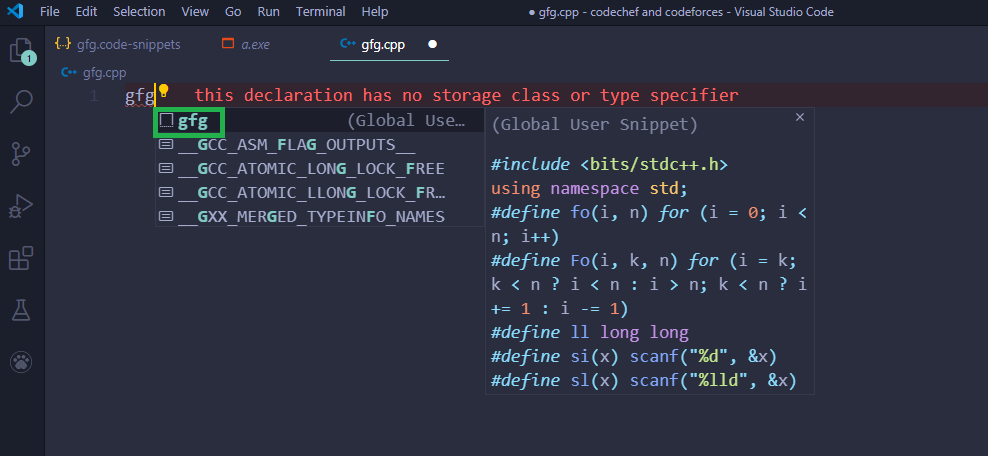• STEP 8: After clicking on the template name which is showing on the screen you will get your entire template.

Screen Set Up

All coding sites use a file comparison method to check answers. It means they store the output through your program in a text file and compare with the actual answer file.

Therefore, you should also do so. What you need to do is create a folder and inside it create 2 files input.txt, output.txt. You should also place your CPP code here. After that, you should configure your editor to a layout shown in the fig: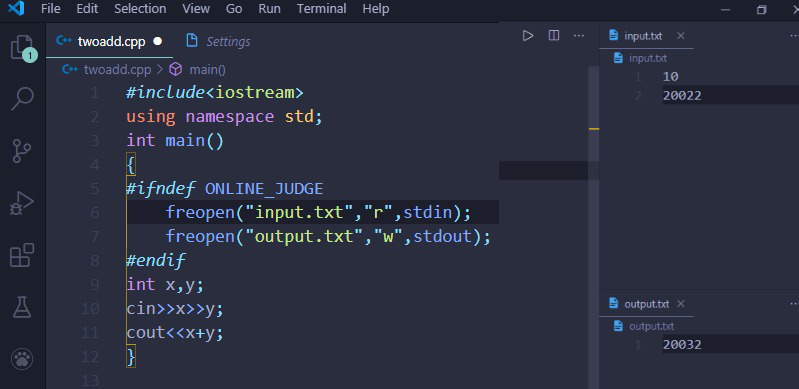To run your code you need to install Code Runner extension in Vscode.

After installing Code Runner just press ALT+CTRL+N to run the code.

Note: When you make any changes in input don’t forgot to save it by pressing ctrl+S in input.txt box.

## C++

 `#ifndef ONLINE_JUDGE ` `freopen``(``"input.txt"``, ``"r"``, stdin); ` `freopen``(``"output.txt"``, ``"w"``, stdout); ` `#endif`

To run CPP code press:

```ALT + CTRL + N
```My Personal Notes arrow_drop_upCheck out this Author's contributed articles.

If you like GeeksforGeeks and would like to contribute, you can also write an article using contribute.geeksforgeeks.org or mail your article to contribute@geeksforgeeks.org. See your article appearing on the GeeksforGeeks main page and help other Geeks.

Please Improve this article if you find anything incorrect by clicking on the "Improve Article" button below.

Article Tags :

5

Please write to us at contribute@geeksforgeeks.org to report any issue with the above content.